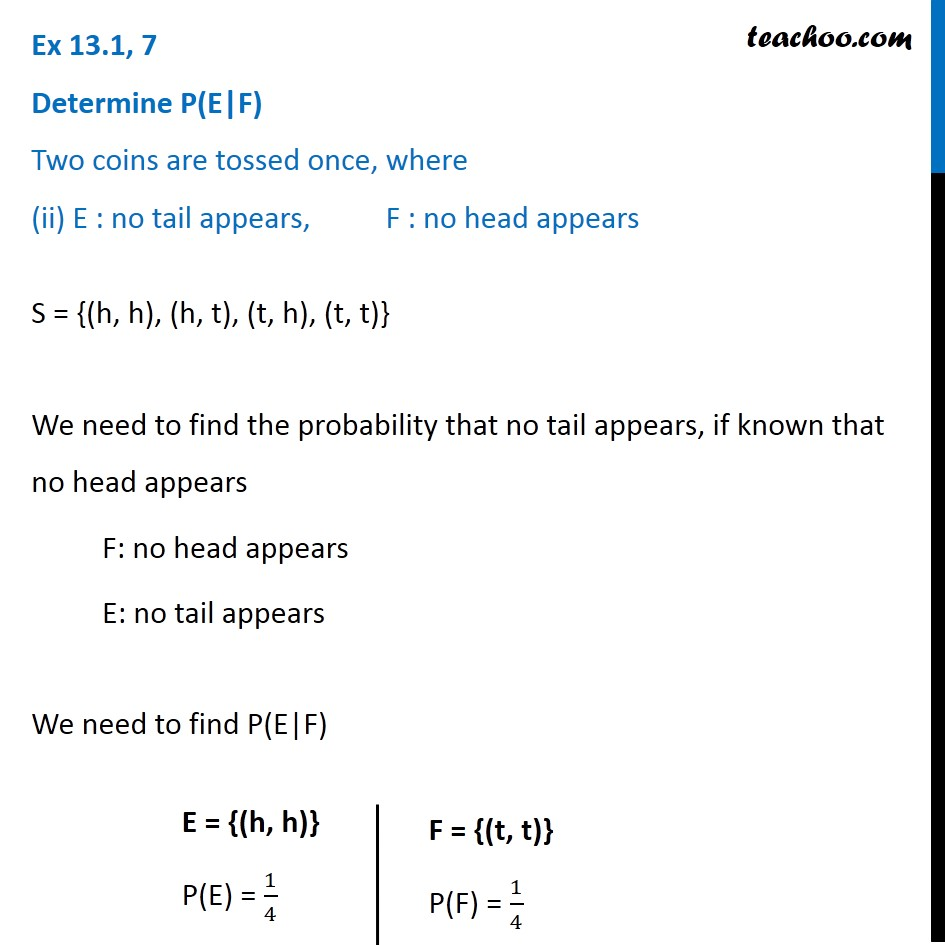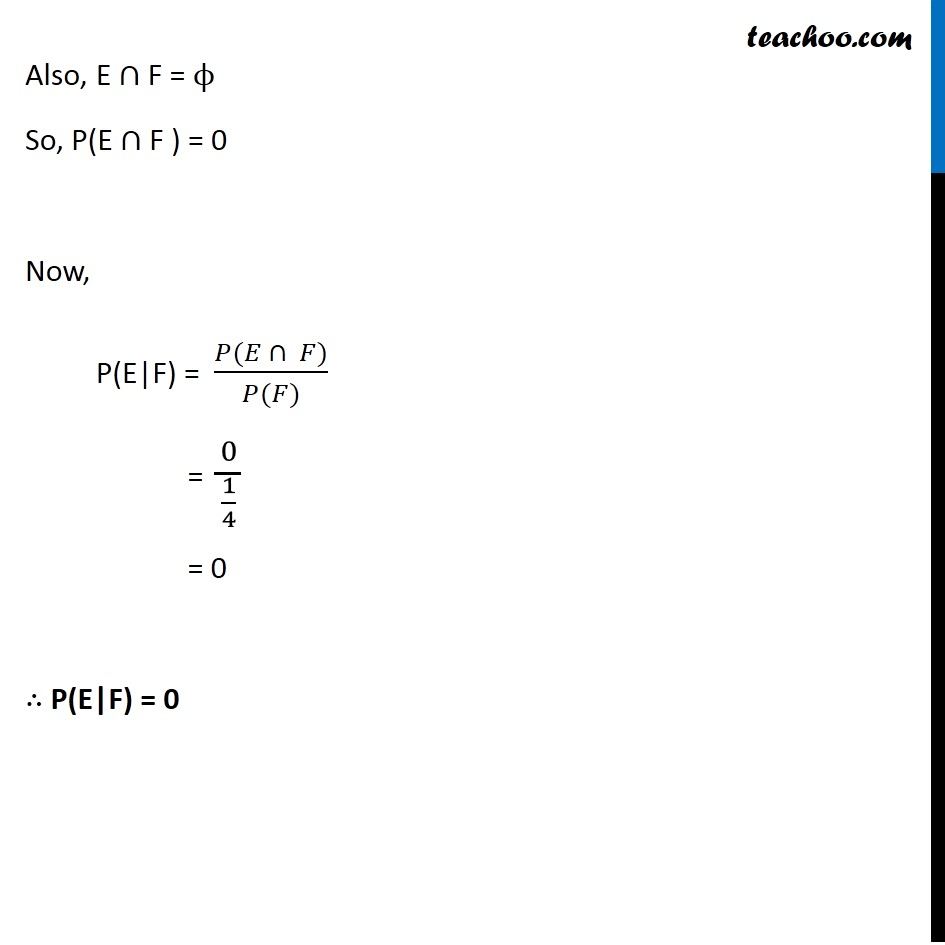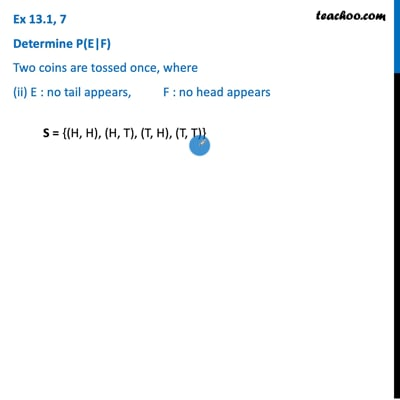Ex 13.1

Chapter 13 Class 12 Probability
Serial order wiseThis video is only available for Teachoo black users

Learn in your speed, with individual attention - Teachoo Maths 1-on-1 Class

### Transcript

Ex 13.1, 7 ﻿Determine P(E|F) Two coins are tossed once, where (ii) E : no tail appears, F : no head appears S = {(h, h), (h, t), (t, h), (t, t)} We need to find the probability that no tail appears, if known that no head appears F: no head appears E: no tail appears We need to find P(E|F) E = {(h, h)} P(E) = 1/4 F = {(t, t)} P(F) = 1/4 Also, E ∩ F = ϕ So, P(E ∩ F ) = 0 Now, P(E|F) = (𝑃(𝐸 ∩ 𝐹))/(𝑃(𝐹)) = 0/(1/4) = 0 ∴ P(E|F) = 0